Open in App
Not now

# Expression Trees Using Classes in C++ with Implementation

• Difficulty Level : Medium
• Last Updated : 25 Nov, 2022

Prerequisite: Expression Tree

The expression tree is a binary tree in which each internal node corresponds to the operator and each leaf node corresponds to the operand so for example expression tree for 3 + ((5+9)*2) would be: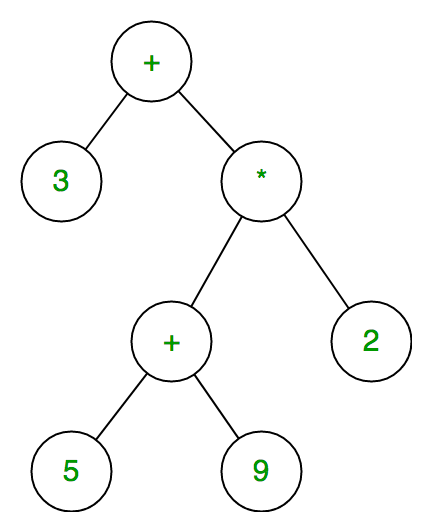In expression trees, leaf nodes are operands and non-leaf nodes are operators. That means an expression tree is a binary tree where internal nodes are operators and leaves are operands. An expression tree consists of binary expressions. But for a unary operator, one subtree will be empty.

Construction of Expression Tree:

• The user will provide a postfix expression for which the program will construct the expression tree.
• Inorder traversal of binary tree/expression tree will provide Infix expression of the given input.

Example:

```Input:  A B C*+ D/
Output: A + B * C / D```

Step 1: The first three symbols are operands, so create tree nodes and push pointers to them onto a stack as shown below.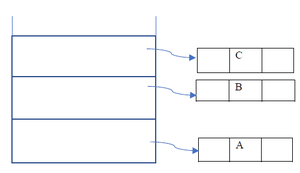Step 2: In the Next step, an operator ‘*’ will going read, so two pointers to trees are popped, a new tree is formed and a pointer to it is pushed onto the stack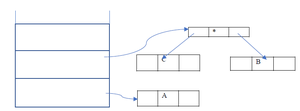Step 3: In the Next step,  an operator ‘+’ will read, so two pointers to trees are popped, a new tree is formed and a pointer to it is pushed onto the stack.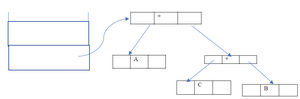Step 4: Similarly, as above cases first we push ‘D’ into the stack and then in the last step first, will read ‘/’ and then as previous step topmost element will pop out and then will be right subtree of root  ‘/’ and other node will be right subtree.

Final Constructed Expression Tree is: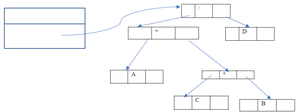Below is the C++ program to implement the above approach:

## C++

 `// C++ program to implement``// the above approach``#include ``using` `namespace` `std;``class` `node {``public``:``    ``char` `value;``    ``node* left;``    ``node* right;``    ``node* next = NULL;``    ``node(``char` `c)``    ``{``        ``this``->value = c;``        ``left = NULL;``        ``right = NULL;``    ``}``    ``node()``    ``{``        ``left = NULL;``        ``right = NULL;``    ``}``    ``friend` `class` `Stack;``    ``friend` `class` `expression_tree;``};` `// Class stack to hold``// tree nodes``class` `Stack {``    ``node* head = NULL;` `public``:``    ``void` `push(node*);``    ``node* pop();``    ``friend` `class` `expression_tree;``};` `// Class to implement``// inorder traversal``class` `expression_tree {``public``:``    ``// Function to implement``    ``// inorder traversal``    ``void` `inorder(node* x)``    ``{``        ``if` `(x == NULL)``            ``return``;``        ``else` `{``            ``inorder(x->left);``            ``cout << x->value << ``"  "``;``            ``inorder(x->right);``        ``}``    ``}``};` `// Function to push values``// onto the stack``void` `Stack::push(node* x)``{``    ``if` `(head == NULL) {``        ``head = x;``    ``}` `    ``// We are inserting nodes at``    ``// the top of the stack [``    ``// following LIFO principle]``    ``else` `{``        ``x->next = head;``        ``head = x;``    ``}``}` `// Function to implement pop``// operation in the stack``node* Stack::pop()``{``    ``// Popping out the top most[``    ``// pointed with head] element``    ``node* p = head;``    ``head = head->next;``    ``return` `p;``}` `// Driver code``int` `main()``{``    ``// Postfix expression``    ``string s = ``"ABC*+D/"``;` `    ``Stack e;``    ``expression_tree a;``    ``node *x, *y, *z;``    ``int` `l = s.length();` `    ``for` `(``int` `i = 0; i < l; i++) {``        ``// if read character is operator``        ``// then popping two other elements``        ``// from stack and making a binary``        ``// tree``        ``if` `(s[i] == ``'+'` `|| s[i] == ``'-'``            ``|| s[i] == ``'*'` `|| s[i] == ``'/'``            ``|| s[i] == ``'^'``) {``            ``z = ``new` `node(s[i]);``            ``x = e.pop();``            ``y = e.pop();``            ``z->left = y;``            ``z->right = x;``            ``e.push(z);``        ``}``        ``else` `{``            ``z = ``new` `node(s[i]);``            ``e.push(z);``        ``}``    ``}` `    ``// Print the inorder traversal``    ``cout << ``" The Inorder Traversal of Expression Tree: "``;``    ``a.inorder(z);``    ``return` `0;``}`

Output:

The Inorder Traversal of Expression Tree: A + B * C / D

My Personal Notes arrow_drop_up